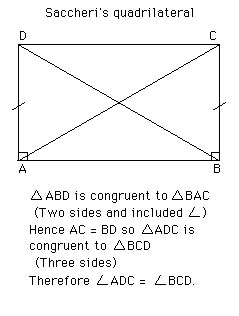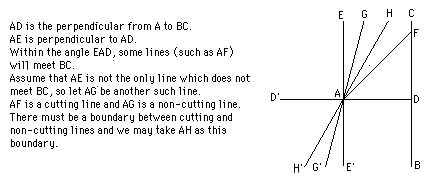# Non-Euclidean geometry

In about 300 BC Euclid wrote The Elements, a book which was to become one of the most famous books ever written. Euclid stated five postulates on which he based all his theorems:
1. To draw a straight line from any point to any other.
2. To produce a finite straight line continuously in a straight line.
3. To describe a circle with any centre and distance.
4. That all right angles are equal to each other.
5. That, if a straight line falling on two straight lines make the interior angles on the same side less than two right angles, if produced indefinitely, meet on that side on which are the angles less than the two right angles.
It is clear that the fifth postulate is different from the other four. It did not satisfy Euclid and he tried to avoid its use as long as possible - in fact the first 28 propositions of The Elements are proved without using it. Another comment worth making at this point is that Euclid, and many that were to follow him, assumed that straight lines were infinite.

Proclus (410-485) wrote a commentary on The Elements where he comments on attempted proofs to deduce the fifth postulate from the other four, in particular he notes that Ptolemy had produced a false 'proof'. Proclus then goes on to give a false proof of his own. However he did give the following postulate which is equivalent to the fifth postulate.
Playfair's Axiom:- Given a line and a point not on the line, it is possible to draw exactly one line through the given point parallel to the line.
Although known from the time of Proclus, this became known as Playfair's Axiom after John Playfair wrote a famous commentary on Euclid in 1795 in which he proposed replacing Euclid's fifth postulate by this axiom.

Many attempts were made to prove the fifth postulate from the other four, many of them being accepted as proofs for long periods of time until the mistake was found. Invariably the mistake was assuming some 'obvious' property which turned out to be equivalent to the fifth postulate. One such 'proof' was given by Wallis in 1663 when he thought he had deduced the fifth postulate, but he had actually shown it to be equivalent to:-
To each triangle, there exists a similar triangle of arbitrary magnitude.
One of the attempted proofs turned out to be more important than most others. It was produced in 1697 by Girolamo Saccheri. The importance of Saccheri's work was that he assumed the fifth postulate false and attempted to derive a contradiction.In this figure Saccheri proved that the summit angles at D and C were equal.The proof uses properties of congruent triangles which Euclid proved in Propositions 4 and 8 which are proved before the fifth postulate is used. Saccheri has shown:
a) The summit angles are > 90° (hypothesis of the obtuse angle).
b
) The summit angles are < 90° (hypothesis of the acute angle).
c
) The summit angles are = 90° (hypothesis of the right angle).

Euclid's fifth postulate is c). Saccheri proved that the hypothesis of the obtuse angle implied the fifth postulate, so obtaining a contradiction. Saccheri then studied the hypothesis of the acute angle and derived many theorems of non-Euclidean geometry without realising what he was doing. However he eventually 'proved' that the hypothesis of the acute angle led to a contradiction by assuming that there is a 'point at infinity' which lies on a plane.

In 1766 Lambert followed a similar line to Saccheri. However he did not fall into the trap that Saccheri fell into and investigated the hypothesis of the acute angle without obtaining a contradiction. Lambert noticed that, in this new geometry, the angle sum of a triangle increased as the area of the triangle decreased.

Legendre spent 40 years of his life working on the parallel postulate and the work appears in appendices to various editions of his highly successful geometry book Eléments de Géométrie . Legendre proved that Euclid's fifth postulate is equivalent to:-
The sum of the angles of a triangle is equal to two right angles.
Legendre showed, as Saccheri had over 100 years earlier, that the sum of the angles of a triangle cannot be greater than two right angles. This, again like Saccheri, rested on the fact that straight lines were infinite. In trying to show that the angle sum cannot be less than 180° Legendre assumed that through any point in the interior of an angle it is always possible to draw a line which meets both sides of the angle. This turns out to be another equivalent form of the fifth postulate, but Legendre never realised his error himself.

Elementary geometry was by this time engulfed in the problems of the parallel postulate. D'Alembert, in 1767, called it the scandal of elementary geometry.

The first person to really come to understand the problem of the parallels was Gauss. He began work on the fifth postulate in 1792 while only 15 years old, at first attempting to prove the parallels postulate from the other four. By 1813 he had made little progress and wrote:
In the theory of parallels we are even now not further than Euclid. This is a shameful part of mathematics...
However by 1817 Gauss had become convinced that the fifth postulate was independent of the other four postulates. He began to work out the consequences of a geometry in which more than one line can be drawn through a given point parallel to a given line. Perhaps most surprisingly of all Gauss never published this work but kept it a secret. At this time thinking was dominated by Kant who had stated that Euclidean geometry is the inevitable necessity of thought and Gauss disliked controversy.

Gauss discussed the theory of parallels with his friend, the mathematician Farkas Bolyai who made several false proofs of the parallel postulate. Farkas Bolyai taught his son, János Bolyai, mathematics but, despite advising his son not to waste one hour's time on that problem of the problem of the fifth postulate, János Bolyai did work on the problem.

In 1823 János Bolyai wrote to his father saying I have discovered things so wonderful that I was astounded ... out of nothing I have created a strange new world. However it took Bolyai a further two years before it was all written down and he published his strange new world as a 24 page appendix to his father's book, although just to confuse future generations the appendix was published before the book itself.

Gauss, after reading the 24 pages, described János Bolyai in these words while writing to a friend: I regard this young geometer Bolyai as a genius of the first order . However in some sense Bolyai only assumed that the new geometry was possible. He then followed the consequences in a not too dissimilar fashion from those who had chosen to assume the fifth postulate was false and seek a contradiction. However the real breakthrough was the belief that the new geometry was possible. Gauss, however impressed he sounded in the above quote with Bolyai, rather devastated Bolyai by telling him that he (Gauss) had discovered all this earlier but had not published. Although this must undoubtedly have been true, it detracts in no way from Bolyai's incredible breakthrough.

Nor is Bolyai's work diminished because Lobachevsky published a work on non-Euclidean geometry in 1829. Neither Bolyai nor Gauss knew of Lobachevsky's work, mainly because it was only published in Russian in the Kazan Messenger a local university publication. Lobachevsky's attempt to reach a wider audience had failed when his paper was rejected by Ostrogradski.

In fact Lobachevsky fared no better than Bolyai in gaining public recognition for his momentous work. He published Geometrical investigations on the theory of parallels in 1840 which, in its 61 pages, gives the clearest account of Lobachevsky's work. The publication of an account in French in Crelle's Journal in 1837 brought his work on non-Euclidean geometry to a wide audience but the mathematical community was not ready to accept ideas so revolutionary.

In Lobachevsky's 1840 booklet he explains clearly how his non-Euclidean geometry works.
All straight lines which in a plane go out from a point can, with reference to a given straight line in the same plane, be divided into two classes - into cutting and non-cutting. The boundary lines of the one and the other class of those lines will be called parallel to the given line.Here is the Lobachevsky's diagram

Hence Lobachevsky has replaced the fifth postulate of Euclid by:-
Lobachevsky's Parallel Postulate. There exist two lines parallel to a given line through a given point not on the line.
Lobachevsky went on to develop many trigonometric identities for triangles which held in this geometry, showing that as the triangle became small the identities tended to the usual trigonometric identities.

Riemann, who wrote his doctoral dissertation under Gauss's supervision, gave an inaugural lecture on 10 June 1854 in which he reformulated the whole concept of geometry which he saw as a space with enough extra structure to be able to measure things like length. This lecture was not published until 1868, two years after Riemann's death but was to have a profound influence on the development of a wealth of different geometries. Riemann briefly discussed a 'spherical' geometry in which every line through a point P not on a line AB meets the line AB. In this geometry no parallels are possible.

It is important to realise that neither Bolyai's nor Lobachevsky's description of their new geometry had been proved to be consistent. In fact it was no different from Euclidean geometry in this respect although the many centuries of work with Euclidean geometry was sufficient to convince mathematicians that no contradiction would ever appear within it.

The first person to put the Bolyai - Lobachevsky non-Euclidean geometry on the same footing as Euclidean geometry was Eugenio Beltrami (1835-1900). In 1868 he wrote a paper Essay on the interpretation of non-Euclidean geometry which produced a model for 2-dimensional non-Euclidean geometry within 3-dimensional Euclidean geometry. The model was obtained on the surface of revolution of a tractrix about its asymptote. This is sometimes called a pseudo-sphere.

You can see the graph of a tractrix at THIS LINK and what the top half of a Pseudo-sphere looks like at THIS LINK.

In fact Beltrami's model was incomplete but it certainly gave a final decision on the fifth postulate of Euclid since the model provided a setting in which Euclid's first four postulates held but the fifth did not hold. It reduced the problem of consistency of the axioms of non-Euclidean geometry to that of the consistency of the axioms of Euclidean geometry.

Beltrami's work on a model of Bolyai - Lobachevsky's non-Euclidean geometry was completed by Klein in 1871. Klein went further than this and gave models of other non-Euclidean geometries such as Riemann's spherical geometry. Klein's work was based on a notion of distance defined by Cayley in 1859 when he proposed a generalised definition for distance.

Klein showed that there are three basically different types of geometry. In the Bolyai - Lobachevsky type of geometry, straight lines have two infinitely distant points. In the Riemann type of spherical geometry, lines have no (or more precisely two imaginary) infinitely distant points. Euclidean geometry is a limiting case between the two where for each line there are two coincident infinitely distant points.

Article by: J J O'Connor and E F Robertson

List of References (23 books/articles)

 JOC/EFR © February 1996 Copyright information School of Mathematics and Statistics University of St Andrews, Scotland